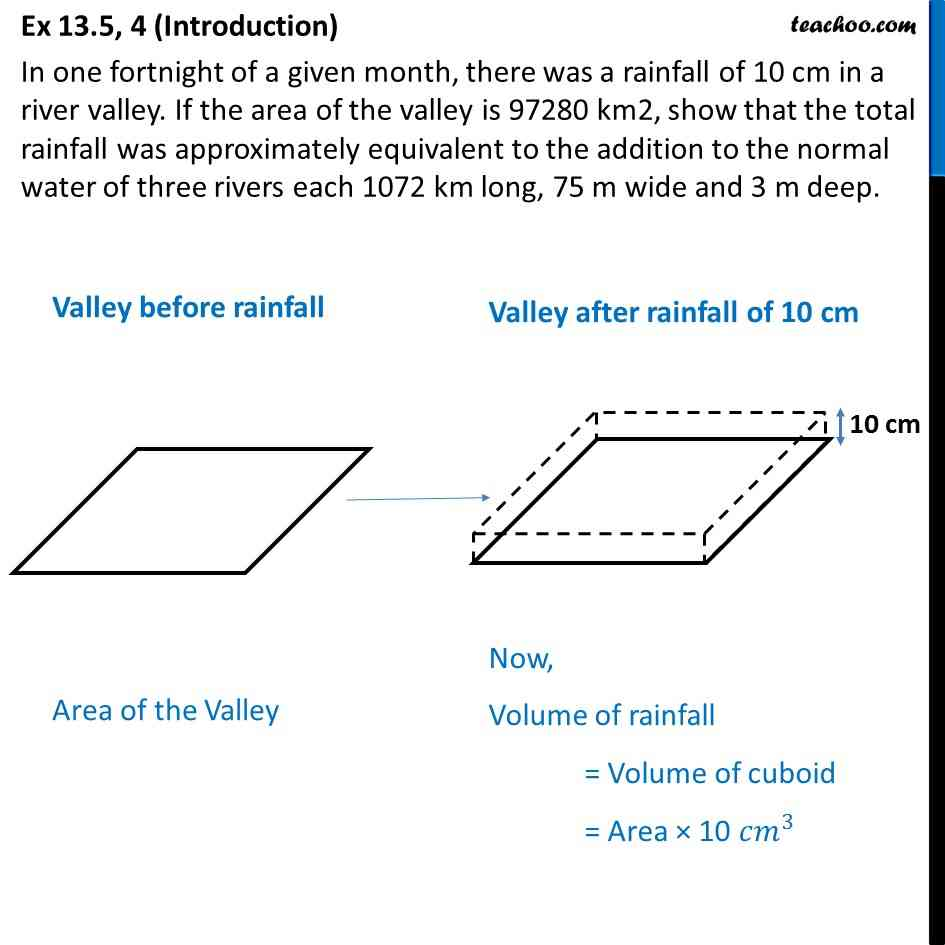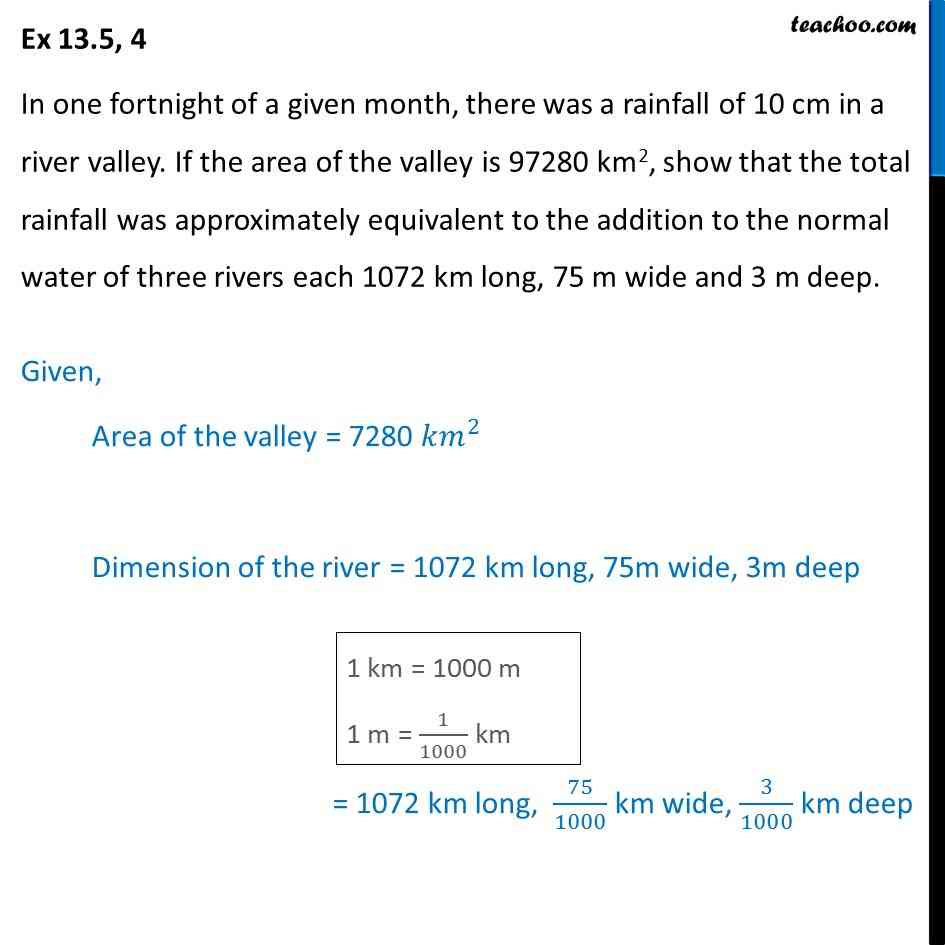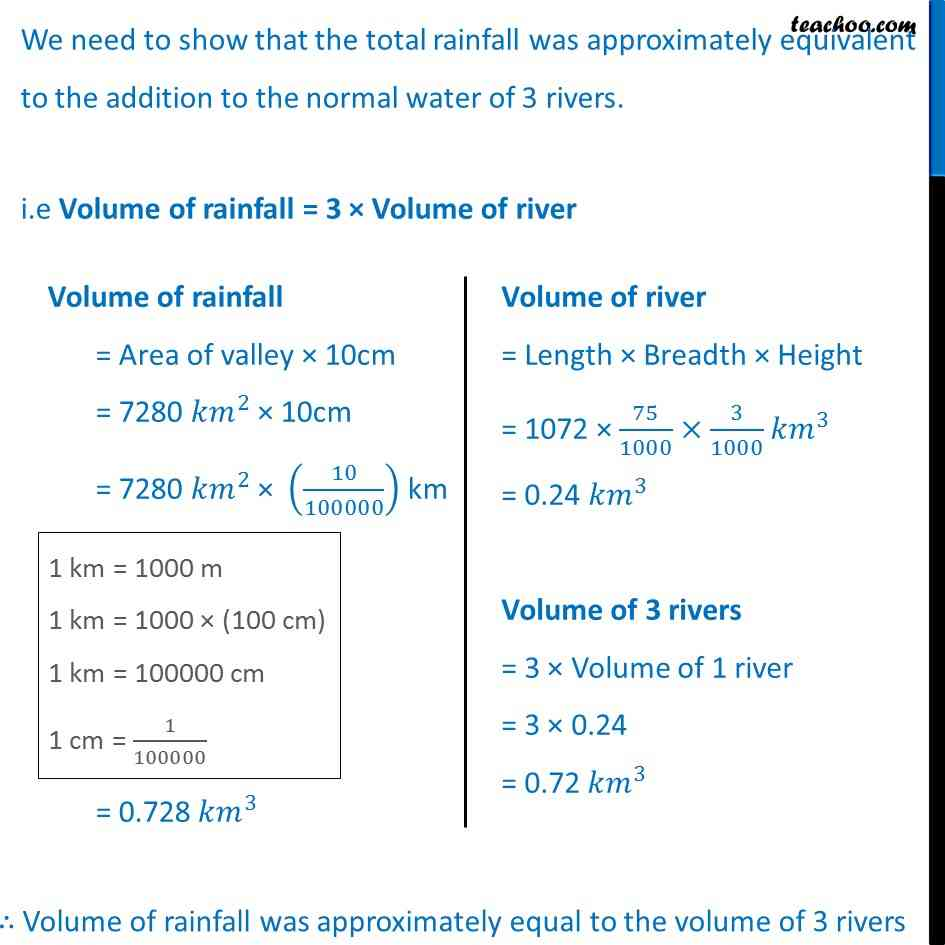Important Questions on Surface Area and Volume

Chapter 12 Class 10 Surface Areas and Volumes
Serial order wiseLearn in your speed, with individual attention - Teachoo Maths 1-on-1 Class

### Transcript

Question 4 (Introduction) In one fortnight of a given month, there was a rainfall of 10 cm in a river valley. If the area of the valley is 97280 km2, show that the total rainfall was approximately equivalent to the addition to the normal water of three rivers each 1072 km long, 75 m wide and 3 m deep. Valley before rainfall Area of the Valley Valley after rainfall of 10 cm Now, Volume of rainfall = Volume of cuboid = Area × 10 〖𝑐𝑚〗^3 Question 4 In one fortnight of a given month, there was a rainfall of 10 cm in a river valley. If the area of the valley is 97280 km2, show that the total rainfall was approximately equivalent to the addition to the normal water of three rivers each 1072 km long, 75 m wide and 3 m deep. Given, Area of the valley = 7280 〖𝑘𝑚〗^2 Dimension of the river = 1072 km long, 75m wide, 3m deep = 1072 km long, 75/1000 km wide, 3/1000 km deep We need to show that the total rainfall was approximately equivalent to the addition to the normal water of 3 rivers. i.e Volume of rainfall = 3 × Volume of river Volume of rainfall = Area of valley × 10cm = 7280 〖𝑘𝑚〗^2 × 10cm = 7280 〖𝑘𝑚〗^2 × (10/100000) km = 0.728〖 𝑘𝑚〗^3 Volume of river = Length × Breadth × Height = 1072 × 75/1000×3/1000 𝑘𝑚^3 = 0.24 𝑘𝑚^3 Volume of 3 rivers = 3 × Volume of 1 river = 3 × 0.24 = 0.72〖 𝑘𝑚〗^3 ∴ Volume of rainfall was approximately equal to the volume of 3 rivers